Processing ......FreeComputerBooks.com Links to Free Computer, Mathematics, Technical Books all over the World

Deep Learning and Neural Networks
Related Book Categories:
 Machine LearningComputer Vision Natural Language Processing (NLP) Big Data Python Programming Statistics and SAS Programming Bayesian Thinking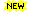Artificial Intelligence (AI) Robotics and Robot ProgrammingData ScienceOptimization and Approximation R Programming
•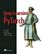Deep Learning with PyTorch (Eli Stevens, et al.)

This book teaches you to create deep learning and neural network systems with PyTorch. It gets you to work right away building a tumor image classifier from scratch. You'll learn best practices for the entire deep learning pipeline, tackling advanced projects.

•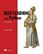Deep Learning with Python, 2nd Edition (Francois Chollet)

This book introduces the field of deep learning using Python and the powerful Keras library. It offers insights for both novice and experienced machine learning practitioners, and builds your understanding through intuitive explanations and practical examples.

•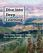Dive into Deep Learning (Aston Zhang, et al.)

This is an open source, interactive book provided in a unique form factor that integrates text, mathematics and code, now supports the TensorFlow, PyTorch, and Apache MXNet programming frameworks, drafted entirely through Jupyter notebooks.

•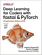Deep Learning for Coders with Fastai and PyTorch

This book show you how to train a model on a wide range of tasks using fastai and PyTorch. You'll also dive progressively further into deep learning theory to gain a complete understanding of the algorithms behind the scenes.

•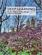Deep Learning (Ian Goodfellow, et al)

Written by three experts, this is the only comprehensive book on the subject. It offers mathematical and conceptual background, covering relevant concepts in linear algebra, probability theory and information theory, numerical computation, and machine learning.

•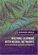Machine Learning with Neural Networks (Bernhard Mehlig)

This modern and self-contained book offers a clear and accessible introduction to the important topic of machine learning with neural networks. It provides comprehensive coverage of neural networks, their evolution, their structure, their applications, etc.

•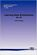Learning Deep Architectures for AI (Yoshua Bengio)

This book discusses the motivations for and principles of learning algorithms for deep architectures. By analyzing and comparing recent results with different learning algorithms for deep architectures, explanations for their success are proposed.

•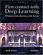First Contact with Deep Learning: Practical Introduction with Keras

This book gradually starts the reader off in Deep Learning, in a practical way with the Python language. Using the Keras library allows the development of Deep Learning models and abstracts much of the mathematical complexity involved in its implementation.

•Neural Networks and Deep Learning (Michael Nielsen)

Neural networks and deep learning currently provide the best solutions to many problems in image recognition, speech recognition, and natural language processing. This book will teach you the core concepts behind neural networks and deep learning.

•Deep Learning: Technical Introduction (Thomas Epelbaum)

This book presents in a technical though hopefully pedagogical way the three most common forms of neural network architectures: Feedforward, Convolutional and Recurrent. For each network, their fundamental building blocks are detailed.

•Neural Network Design (Martin T. Hagan)

This book provides a clear and detailed coverage of fundamental neural network architectures and learning rules. It emphasizes a coherent presentation of the principal neural networks, methods for training them and their applications to practical problems.

•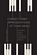Discovering Musical Patterns by Artificial Neural Networks

Intended to introduce readers to the use of Artificial Neural Networks in the study of music, this book contains numerous case studies and research findings that address problems related to identifying scales, keys, classifying musical chords, etc.

•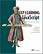Deep Learning with JavaScript: Neural Networks in TensorFlow.js

This book shows developers how they can bring Deep Learning technology to the web. Written by the main authors of the TensorFlow library, it provides fascinating use cases and in-depth instruction for deep learning apps in JavaScript in your browser or on Node.

•GANs in Action: Learning with Generative Adversarial Networks

This book teaches you how to build and train your own Generative Adversarial Networks, one of the most important innovations in deep learning. You'll learn how to start building your own simple adversarial system as you explore the foundation of GAN architecture.

•Deep Learning and the Game of Go (Max Pumperla, et al)

This book teaches you how to apply the power of deep learning to complex reasoning tasks by building a Go-playing AI. After exposing you to the foundations of machine and deep learning, you'll use Python to build a bot and then teach it the rules of the game.

•A Survey of Statistical Network Models (Anna Goldenberg, et al.)

This book aims to provide the reader with an entry point to the voluminous literature on statistical network modeling. It guides the reader through the development of key stochastic network models, touches upon a number of examples and commonalities.

•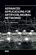Advanced Applications for Artificial Neural Networks (Adel E.)

In this book, highly qualified multidisciplinary scientists grasp their recent researches motivated by the importance of Artificial Neural Networks (ANN). It addresses advanced applications and innovative case studies for different fields using ANN.

•Neural Networks with JavaScript Succinctly (James McCaffrey)

This book leads you through the fundamental concepts of neural networks, including its architecture, its input-output, tanh and softmax activation, back-propagation, error and accuracy, normalization and encoding, and model interpretation.

•Deep Learning Tutorials (LISA Lab)

The tutorials presented here will introduce you to some of the most important deep learning algorithms and will also show you how to run them using Theano. Theano is a python library that makes writing deep learning models easy, and gives the option of training them on a GPU.

•Deep Learning in Neural Networks: An Overview (J. Schmidhuber)

It compactly summarises relevant work, much of it from the previous millennium. Shallow and deep learners are distinguished by the depth of their credit assignment paths, which are chains of possibly learnable, causal links between actions and effects.

•Machine Learning, Neural and Statistical Classification (D. Michie)

Statistical, machine learning and neural network approaches to classification are all covered in this book to provide an objective assessment of the potential for machine learning algorithms in solving significant commercial and industrial problems, widening the foundation for exploitation of these and related algorithms.

•Applied Artificial Neural Networks (Christian Dawson)

This book focuses on the application of neural networks to a diverse range of fields and problems. It collates contributions concerning neural network applications in areas such as engineering, hydrology and medicine.

•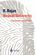Neural Networks - A Systematic Introduction (Raul Rojas)

In this book, theoretical laws and models previously scattered in the literature are brought together into a general theory of artificial neural nets. It is aimed at readers who seek an overview of the field or who wish to deepen their knowledge.

•An Introduction to Neural Networks (Kevin Gurney)

With an easy to understand format using graphical illustrations and multidisciplinary scientific context, this book fills the gap in the market for neural networks for multi-dimensional scientific data, and relates neural networks to statistics.

•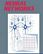Neural Networks (Rolf Pfeifer, et al)

Beginning with an introductory discussion on the role of neural networks in scientific data analysis, this book provides a solid foundation of basic neural network concepts. It is a systematic introduction to neural networks, biological foundation.

•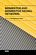Memristor and Memristive Neural Networks (Alex James)

This book covers a range of models, circuits and systems built with memristor devices and networks in applications to neural networks. It is divided into three parts: (1) Devices, (2) Models and (3) Applications.

•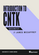Introduction to CNTK (Microsoft Cognitive Toolkit) Succinctly

This book will be a quick introduction to using Cognitive Toolkit and will teach you how to train and validate different types of neural networks, such as convolutional and recurrent neural networks. It offers instruction on the basics of installing and running CNTK.

•Keras Succinctly (James McCaffrey)

The goal of this book is to introduce you Keras, the one of the most popular and powerful libraries for building neural networks in Python. You'll learn how to build a convolutional neural network in Python!

•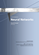A Brief Introduction to Neural Networks (David Kriesel)

Introduces the Java programmer to the world of Neural Networks and Artificial Intelligence using SNIPE. Examples include the traveling salesman problem, handwriting recognition, financial prediction, game strategy, mathematical functions, and Internet bots.

•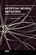Artificial Neural Networks - Models and Applications

This is a current book on Artificial Neural Networks and Applications, bringing recent advances in the area to the reader interested in this always-evolving machine learning technique. It contains chapters on basic concepts of artificial neural networks.

•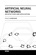Artificial Neural Networks - Architectures and Applications

This book covers architectures, design, optimization, and analysis of artificial neural networks as well as applications of artificial neural networks in a wide range of areas including biomedical, industrial, physics, and financial applications.

•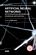Artificial Neural Networks - Methodological Advances and Apps

The book begins with fundamentals of artificial neural networks, which cover an introduction, design, and optimization. Advanced architectures for biomedical applications, which offer improved performance and desirable properties, follow.

•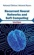Recurrent Neural Networks and Soft Computing (M. ElHefnawi)

Advanced information regarding the theory, concepts and applications of recurrent neural networks and the field of soft computing has been highlighted in this elaborative book. Additional topics in this vein are the application of AI techniques to electromagnetic interference problems, etc.

•Recurrent Neural Networks for Temporal Data Processing

By presenting the latest research work the book demonstrates how real-time recurrent neural networks (RNNs) can be implemented to expand the range of traditional signal processing techniques and to help combat the problem of prediction.

•Recurrent Neural Networks (Xiaolin Hu, et al)

This book investigates the following Recurrent Neural Networks (RNNs) models which solve some practical problems, together with their corresponding analysis on stability and convergence.

•Neural Network Toolbox for MATLAB (Howard Demuth, et al)

It provides algorithms, functions, and apps to create, train, visualize, and simulate neural networks. You can perform classification, regression, clustering, dimensionality reduction, time-series forecasting, and dynamic system modeling and control.

•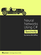Neural Networks Using C# Succinctly (James McCaffrey)

This book teaches you how to create your own neural network to solve classification problems, or problems where the outcomes can only be one of several values.

•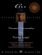C++ Neural Networks and Fuzzy Logic (Valluru B. Rao, et al)

Provides a logical and easy-to-follow progression through C++ programming for two of the most popular technologies for artificial intelligence: neural and fuzzy programming.

•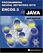Programming Neural Networks with Encog3 in Java (Jeff Heaton)

This book focuses on using the neural network capabilities of Encog with the Java programming language. It begins with an introduction to the kinds of tasks neural networks are suited towards.

•Introduction to Neural Networks with Java (Jeff Heaton)

This book introduces the Java programmer to the world of Neural Networks and Artificial Intelligence. Neural network architectures such as the feedforward backpropagation, Hopfield, and Kohonen networks are discussed.

•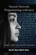Neural Network Programming with Java (Alan M.F. Souza, et al)

This book gives you a complete walkthrough of the process of developing basic to advanced practical examples based on neural networks with Java. No previous knowledge of neural networks is required as this book covers the concepts from scratch.

Book Categories
 :All CategoriesRecent BooksMiscellaneous BooksComputer LanguagesComputer ScienceData Science/DatabasesElectrical EngineeringJava and Java EE (J2EE)Linux and UnixMathematicsMicrosoft and .NETMobile ComputingNetworking and CommunicationsSoftware EngineeringSpecial TopicsWeb Programming
Other Categories